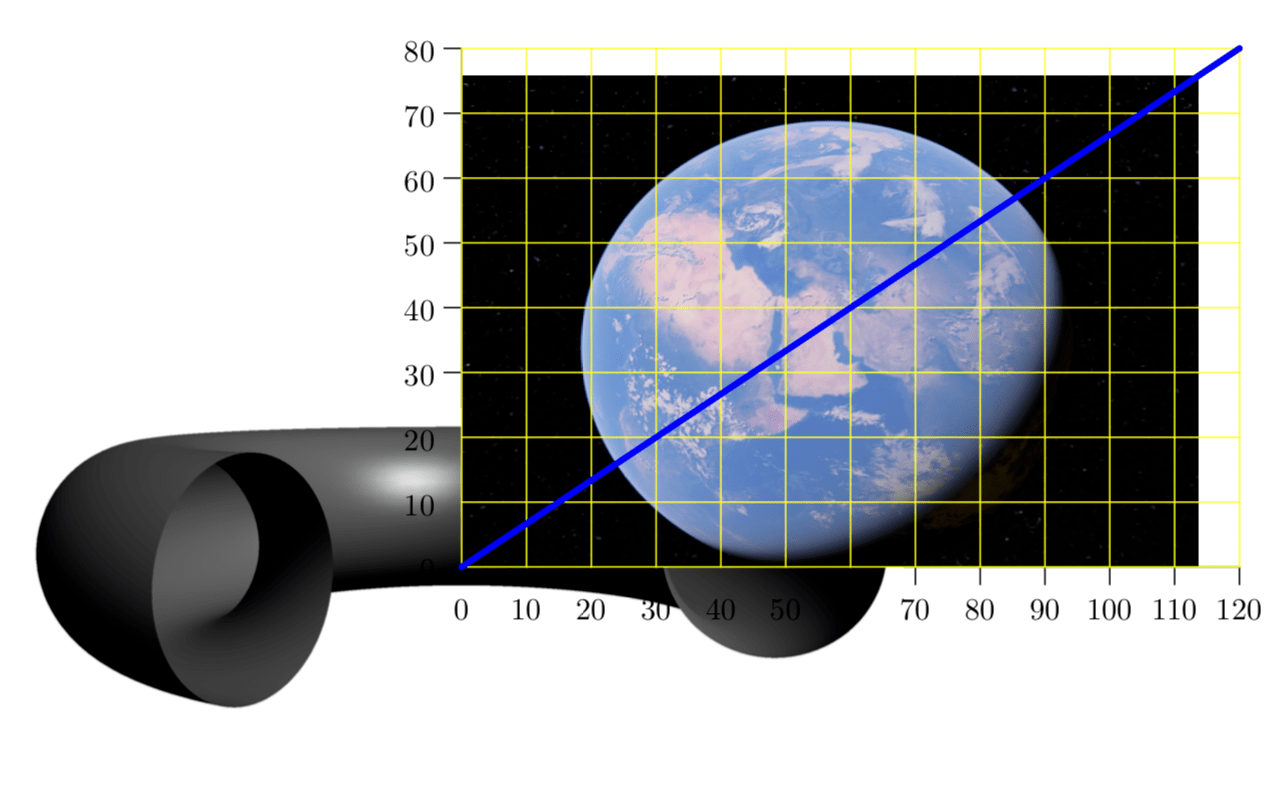# #StackBounty: #asymptote Drawing 3D graphics on top of an imported picture with asymptote

### Bounty: 500

I am trying to draw stuff on top of an imported picture with `asymptote`, following this nice answer. Here is my MWE

``````     documentclass{article}
usepackage[inline]{asymptote}
begin{document}
begin{asy}
size(10cm);
import graph3;
import three;
import labelpath3;
import graph;
import math;

// it is not important which precise picture gets imported
defaultpen(fontsize(10pt));
real sc=2;
unitsize(sc*1bp);
real wd=120*sc;
real ht=80*sc;
label(
shift(wd/2,ht/2)*
graphic("earth.pdf"
,"width="+string(wd)+"bp"
+",height="+string(ht)+"bp"
+",scale="+string(sc)
),(0,0)
);
layer();

int ngrid=10;
int n=(int)(wd/ngrid/sc);
int m=(int)(ht/ngrid/sc);

xaxis( 0,wd/sc,RightTicks(Step=ngrid));
yaxis(0,ht/sc,LeftTicks(Step=ngrid));

draw(((0,0)--(wd,ht)/sc),blue+2pt);

triple f(real t) {
return (3*cos(0.125*2pi*t)+0.08*cos(2pi*t), 3*sin(0.125*2pi*t),0+ 0.08*sin(2pi*t));
}

path3 helix = graph(f, 0, 8, n=500, operator..);

surface helixtube = tube(helix, width=0.4).s;

draw(helixtube, surfacepen=material(blue+opacity(0.3), emissivepen=0.2*white));

real R=300;
real a=100;

triple f(pair t) {
return ((R+a*cos(t.y))*cos(t.x),(R+a*cos(t.y))*sin(t.x),a*sin(t.y));
}

draw(s,gray,render(compression=Low,merge=true));

// ---

draw(((0,0)--(wd,ht)/sc),blue+2pt);

end{asy}
end{document}
endinput
``````

It producesAs one can see, the 2-dimensional stuff, i.e. the grid and the blue line, really appears in front of the picture. However, the 3-dimensional stuff, i.e. the torus, does not. (No, of course I am not expecting `asymptote` to recognize that the imported stuff is also 3-dimensional.) I’d like to see the 3D stuff also in front of the imported picture. Is that possible?

(Of course, in the best of all worlds, somebody might figure out how to draw a 3D earth with e.g. `Mathematica`s GeoData in `asymptote`. Here I just want to put the 3D stuff in front of the background picture. It would be great if `opacity` would also work…)

Get this bounty!!!

This site uses Akismet to reduce spam. Learn how your comment data is processed.